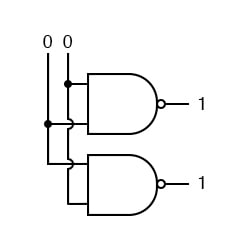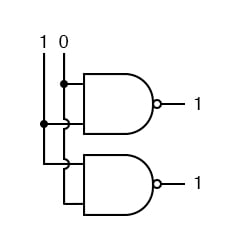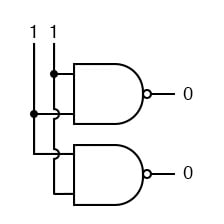# Introduction to Combinational Logic Functions

## Chapter 9 - Combinational Logic Functions

The term “combinational” comes to us from mathematics. In mathematics a combination is an unordered set, which is a formal way to say that nobody cares which order the items came in. Most games work this way, if you rolled dice one at a time and get a 2 followed by a 3 it is the same as if you had rolled a 3 followed by a 2. With combinational logic, the circuit produces the same output regardless of the order the inputs are changed.

There are circuits which depend on when the inputs change, these circuits are called sequential logic. Even though you will not find the term “sequential logic” in the chapter titles, the next several chapters will discuss sequential logic.

Practical circuits will have a mix of combinational and sequential logic, with sequential logic making sure everything happens in order and combinational logic performing functions like arithmetic, logic, or conversion.

You have already used combinational circuits. Each logic gate discussed previously is a combinational logic function. Let’s follow how two NAND gate works if we provide them inputs in different orders.

We begin with both inputs being 0.We then set one input high.We then set the other input high.So NAND gates do not care about the order of the inputs, and you will find same behavior to all the other gates covered up to this point (AND, XOR, OR, NOR, XNOR, and NOT).

RELATED WORKSHEET:

• Share
Published under the terms and conditions of the Design Science License